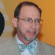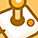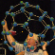cancel
Showing results for
Did you mean:New Contributor

I'm peeping the NIST thermo tables for H and H2, and I'm perplexed.

Seems to me that using G=[ΔHf+H−H(Tr)]−T⋅S (well, actually correcting the entropy for partial pressures in a mixture of ideal gases) obtains some bewildering values for simple reactions. Consider H2→2H at standard pressure and standard temperature. If I'm calculating it correctly (which I am sure I am not), using the values at 298.15∘K in columns 2 (S), 4 (H−H(Tr)), and 5 (ΔHf) from the links above, I find that

G_H2= 0 kJ mol^-1 − 298.15 K⋅130.680 kJ K^-1 mol^-1 ≈ −38962 kJ mol^-1

and

G_H= 217.999 kJ mol^-1 − 298.15 K⋅114.716 kJ K^-1 mol^-1 ≈ −33984 kJ mol^-1

Which is great, the reaction isn't spontaneous. Except... if I recall correctly, G_mixture = ∑ μj nj, where nj is the number of mols of that species and for ideal gases at constant pressure & temperature, μj = Hj − Tsj = Gj.

That would imply the mixture of one mol of H2has a total gibbs of the aformentioned −38962 kJ/mol , but the mixture of two mols of H (pressure neglected) would be 2 × (−33984 kJ/mol)= −67969 kJ/mol, and now supposedly I've found that hydrogen gas spontaneously decomposes at standard temperature. That's clearly wrong.

(I've even done the calculations for a reaction considering pressure, and the doubling of mols of mixture post-reaction isn't enough to drive it into non-spontaneity except at absurdly high pressures)

So my question is: do I misunderstand how the Gibbs works for mixture of gases, how to read values off of the NIST themo tables, or both?

Thank you!

4 RepliesContributor III

## Re: Question about reading NIST datasheets & Gibbs energy of reaction

Dear Anton,

I'm not sure about rechecking all of your assumptions and calculations.  However, I did find this resource (Khan Academy) to provide a much clearer explanation of what Gibbs Energy is all about.  Maybe it will also give you some idea of what may be missing in your exercise.

Best regards,

StevenNew Contributor II

## Re: Question about reading NIST datasheets & Gibbs energy of reaction

Anton--

Are you sure the entropy values are in kJ mol-1 K-1 rather than in J mol-1 K-1?

130.680 kJ K^-1 mol^-1 is a lot of entropy.

--David ShobeContributor

## Re: Question about reading NIST datasheets & Gibbs energy of reaction

David Shope has pointed out the answer. The units of heat capacity and entropy are J/(mol K).  Units matter!

Needless to say, the cleavage of H-H to 2H is endothermic by exactly the H-H bond energy: 2x 218.0 kJ/mol = 436.0 kJ/mol. The entropy effect is small at room temperature. This bond cleavage is endergonic by 2x 203.3 kJ/mol.